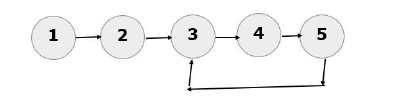# Python Program To Detect A Loop In A Linked List

A linked list is said to have a loop when any node in the linked list is not pointing to NULL. The last node will be pointing to one of the previous nodes in the linked list, thus creating a loop. There will not be an end in a linked list that has a loop.

In the below example, the last node (node 5) is not pointing to NULL. Instead, it is pointing to the node 3 and a loop is established. Hence, there is no end to the above linked list.## Algorithm Take two pointers fast and slow

• Both the pointer will be initially pointing towards the HEAD of the linked list.

• The slow pointer will always be incremented by one and the fast pointer will always be incremented by two.

• At any point, if both the fast pointer and the slow pointer are pointing towards the same node, then the linked list is said to have a loop.

Consider the below example of a linked list where the last node is pointing towards the second node −

### Example

Both the slow pointer and the fast pointer are pointing towards the same node. Hence, it can be concluded that the given linked list contains a loop.

class Node:
def __init__(self, val):
self.val = val
self.next = None

def __init__(self):

def insert_at_the_end(self,newVal):
newNode=Node(newVal)
return
while(temp.next):
temp=temp.next
temp.next=newNode

def Print_the_LL(self):
if(temp != None):
print("\nThe linked list elements are:", end=" ")
while (temp != None):
print(temp.val, end=" ")
temp = temp.next
else:
print("The list is empty.")
def detect_loop(self):
while(fast):
if slow==fast:
print("\nA loop has been detected in the linked list ")
return
slow=slow.next
fast=fast.next

newList.insert_at_the_end(1)
newList.insert_at_the_end(2)
newList.insert_at_the_end(3)
newList.insert_at_the_end(4)
newList.Print_the_LL()
print("\n")
newList.detect_loop()


### Output

A loop has been detected in the linked list.

The linked list elements are: 1 2 3 4

A loop has been detected in the linked list


Updated on: 24-Apr-2023

302 Views